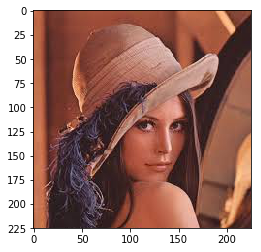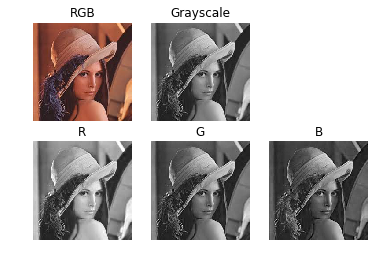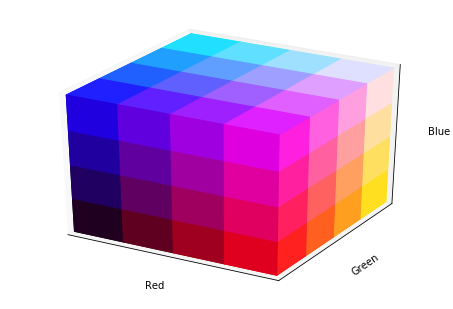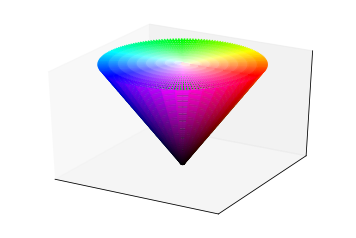Notebook

# Digital Image Processing

In :
% run load_lib_ch0.py


## Introduction: What is a digital image?

Image Processing is the use of algorithms to perform operations on a digital image in order to achieve a particular result such as noise removal or feature extraction. In this notebook, various Digital Image Processing (DIP) techniques will be discussed and demonstrated. The topics covered will be explained as simply as possible and more references will be provided for those who want to learn in more details. Many concepts will be shown using Python. The code can used in each chapter can be seen by clicking on the link in the chapter description, such as the one here.

Play around with the codes to better understand the topics!

Also make sure you check out this awesome book on Computer Vision: Algorithms and Applications by Richard Szeliski!

#### What is a digital image?

A digital image is a two dimensional array of pixels represented as a function of the x- and y-axes, $f(x,y)$. The function $f(x,y)$ returns the value of the pixel intensity at coordinates x and y. Notice that the $(0,0)$ coordinates of the image below are in the top-left corner rather than bottom left as in typical Cartesian coordinates.

In :
plt.imshow(img)
plt.show()A pixel is the smallest element of an image. The snippet can be used to show the pixel value at different coordinates. Each pixels is either a signle value or a vector of values depending on the used colour model. A colour image is essentially just multiple grayscale layers, such as the rgb-image function $f(x,y)=[r(x,y),g(x,y),y(x,y)]$

In :
x = 10
y = 12

In :
select_pixel(img, x,y)Out:
'The pixel at these coordinates (10, 12) is R: 113 G: 51 B: 38'

A colour model is the mathematical representation of colours as groups of number values.The various modules have different benefits and drawbacks that can be used for specific purposes.

Single channel models are unable to describe colour, and contain a single channel that describes the luminosity:

• Binary
• Grayscale

Most common colour models consist of three channels. Each channel contains different information:

• RGB, Red Green Blue
• HSV, Hue Saturation Value
• YUV, Luminace Chroma Chroma

There are some four channel models:

• RGBA, Red Green Blue Alpha
• CMYK, Cyan Magenta Yellow Black

Individually each channel can be represented as a grayscale image, but when combined following their model a colour image can be generated.

In :
display_layers(img)#### Colour Models as Shapes

Three channel colour models can be represented as 3D shapes that better show the relationship between each channel.

In :
rgb_color_model()RGB is an additive colour model, and as such it is shaped as a cube. The whitest colour is a combination of maximum intensity R,G and B.

In :
hsv_color_model()HSV is not additive because the colour information is stored within the Hue layer only. Hue depends on the angle relative to the x/y axes, increase in intensity is shown as the distance from the origin point to the colour. Saturation is the distance from the centre pole (z-axis).

The whitest colour is one with maximum value and minimum saturation, independent of hue.